# Title 40

## SECTION 53.55

### 53.55 Test for effect of variations in power line voltage and ambient temperature.

§ 53.55 Test for effect of variations in power line voltage and ambient temperature.

(a) Overview. (1) This test procedure is a combined procedure to test various performance parameters under variations in power line voltage and ambient temperature. Tests shall be conducted in a temperature-controlled environment over four 6-hour time periods during which reference temperature and flow rate measurements shall be made at intervals not to exceed 5 minutes. Specific parameters to be evaluated at line voltages of 105 and 125 volts and temperatures of −20 °C and + 40 °C are as follows:

(i) Sample flow rate.

(ii) Flow rate regulation.

(iii) Flow rate measurement accuracy.

(iv) Coefficient of variability measurement accuracy.

(v) Ambient air temperature measurement accuracy.

(vi) Proper operation of the sampler when exposed to power line voltage and ambient temperature extremes.

(2) The performance parameters tested under this procedure, the corresponding minimum performance specifications, and the applicable test conditions are summarized in table E-1 of this subpart. Each performance parameter tested, as described or determined in the test procedure, must meet or exceed the associated performance specification given. The candidate sampler must meet all specifications for the associated PM 2.5 or PM 10-2.5 method (as applicable) to pass this test procedure.

(b) Technical definition. Sample flow rate means the quantitative volumetric flow rate of the air stream caused by the sampler to enter the sampler inlet and pass through the sample filter, measured in actual volume units at the temperature and pressure of the air as it enters the inlet.

(c) Required test equipment. (1) Environmental chamber or other temperature-controlled environment or environments, capable of obtaining and maintaining temperatures at −20 °C and = 40 °C as required for the test with an accuracy of ±2 °C. The test environment(s) must be capable of maintaining these temperatures within the specified limits continuously with the additional heat load of the operating test sampler in the environment. Henceforth, where the test procedures specify a test or environmental “chamber,” an alternative temperature-controlled environmental area or areas may be substituted, provided the required test temperatures and all other test requirements are met.

(2) Variable voltage AC power transformer, range 100 Vac to 130 Vac, with sufficient current capacity to operate the test sampler continuously under the test conditions.

(3) Flow rate meter, suitable for measuring and recording the actual volumetric sample flow rate at the sampler downtube, with a minimum range of 10 to 25 actual L/min, 2 percent certified, NIST-traceable accuracy. Optional capability for continuous (analog) recording capability or digital recording at intervals not to exceed 5 minutes is recommended. While a flow meter which provides a direct indication of volumetric flow rate is preferred for this test, an alternative certified flow measurement device may be used as long as appropriate volumetric flow rate corrections are made based on measurements of actual ambient temperature and pressure conditions.

(4) Ambient air temperature recorder, range −30 °C to = 50 °C, with a resolution of 0.1 °C and certified accurate to within 0.5 °C. Ambient air temperature measurements must be made using continuous (analog) recording capability or digital recording at intervals not to exceed 5 minutes.

(5) Barometer, range 600 mm Hg to 800 mm Hg, certified accurate to 2 mm Hg. If the certified flow rate meter does not provide direct volumetric flow rate readings, ambient pressure measurements must be made using continuous (analog) recording capability or digital recording at intervals not to exceed 5 minutes.

(6) Flow measurement adaptor (40 CFR part 50, appendix L, figure L-30) or equivalent adaptor to facilitate measurement of sampler flow rate at the sampler downtube.

(7) Means for creating an additional pressure drop of 55 mm Hg in the sampler to simulate a heavily loaded filter, such as an orifice or flow restrictive plate installed in the filter holder or a valve or other flow restrictor temporarily installed in the flow path near the filter.

(8) AC RMS voltmeter, accurate to 1.0 volt.

(9) Teflon sample filter, as specified in section 6 of 40 CFR part 50, appendix L (if required).

(d) Calibration of test measurement instruments. Submit documentation showing evidence of appropriately recent calibration, certification of calibration accuracy, and NIST-traceability (if required) of all measurement instruments used in the tests. The accuracy of flow rate meters shall be verified at the highest and lowest pressures and temperatures used in the tests and shall be checked at zero and at least one flow rate within ±3 percent of 16.7 L/min within 7 days prior to use for this test. Where an instrument's measurements are to be recorded with an analog recording device, the accuracy of the entire instrument-recorder system shall be calibrated or verified.

(e) Test setup. (1) Setup of the sampler shall be performed as required in this paragraph (e) and otherwise as described in the sampler's operation or instruction manual referred to in § 53.4(b)(3). The sampler shall be installed upright and set up in the temperature-controlled chamber in its normal configuration for collecting PM samples. A sample filter and (or) the device for creating an additional 55 mm Hg pressure drop shall be installed for the duration of these tests. The sampler's ambient temperature, ambient pressure, and flow measurement systems shall all be calibrated per the sampler's operating manual within 7 days prior to this test.

(2) The inlet of the candidate sampler shall be removed and the flow measurement adaptor installed on the sampler's downtube. A leak check as described in the sampler's operation or instruction manual shall be conducted and must be properly passed before other tests are carried out.

(3) The inlet of the flow measurement adaptor shall be connected to the outlet of the flow rate meter.

(4) The ambient air temperature recorder shall be installed in the test chamber such that it will accurately measure the temperature of the air in the vicinity of the candidate sampler without being unduly affected by the chamber's air temperature control system.

(f) Procedure. (1) Set up the sampler as specified in paragraph (e) of this section and otherwise prepare the sampler for normal sample collection operation as directed in the sampler's operation or instruction manual.

(2) The test shall consist of four test runs, one at each of the following conditions of chamber temperature and electrical power line voltage (respectively):

(i) −20 °C ±2 °C and 105 ±1 Vac.

(ii) −20 °C ±2 °C and 125 ±1 Vac.

(iii) = 40 °C ±2 °C and 105 ±1 Vac.

(iv) = 40 °C ±2 °C and 125 ±1 Vac.

(3) For each of the four test runs, set the selected chamber temperature and power line voltage for the test run. Upon achieving each temperature setpoint in the chamber, the candidate sampler and flow meter shall be thermally equilibrated for a period of at least 2 hours prior to the test run. Following the thermal conditioning time, set the sampler to automatically start a 6-hour sample collection period at a convenient time.

(4) During each 6-hour test period:

(i) Measure and record the sample flow rate with the flow rate meter at intervals not to exceed 5 minutes. If ambient temperature and pressure corrections are necessary to calculate volumetric flow rate, ambient temperature and pressure shall be measured at the same frequency as that of the certified flow rate measurements. Note and record the actual start and stop times for the 6-hour flow rate test period.

(ii) Determine and record the ambient (chamber) temperature indicated by the sampler and the corresponding ambient (chamber) temperature measured by the ambient temperature recorder specified in paragraph (c)(4) of this section at intervals not to exceed 5 minutes.

(iii) Measure the power line voltage to the sampler at intervals not greater than 1 hour.

(5) At the end of each test run, terminate the sample period (if not automatically terminated by the sampler) and download all archived instrument data from the test sampler.

(g) Test results. For each of the four test runs, examine the chamber temperature measurements and the power line voltage measurements. Verify that the temperature and line voltage met the requirements specified in paragraph (f) of this section at all times during the test run. If not, the test run is not valid and must be repeated. Determine the test results as follows:

(1) Mean sample flow rate. (i) From the certified measurements (Qref) of the test sampler flow rate, tabulate each flow rate measurement in units of L/min. If ambient temperature and pressure corrections are necessary to calculate volumetric flow rate, each measured flow rate shall be corrected using its corresponding temperature and pressure measurement values. Calculate the mean flow rate for each sample period (Qref,ave) as follows:

Equation 11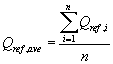where: n equals the number of discrete certified flow rate measurements over each 6-hour test period.

(ii)(A) Calculate the percent difference between this mean flow rate value and the design value of 16.67 L/min, as follows:

Equation 12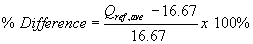(B) To successfully pass this test, the percent difference calculated in Equation 12 of this paragraph (g)(1)(ii) must be within ±5 percent for each test run.

(2) Sample flow rate regulation. (i) From the certified measurements of the test sampler flow rate, calculate the sample coefficient of variation of the discrete measurements as follows:

Equation 13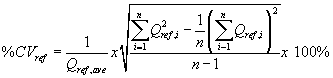(ii) To successfully pass this test, the calculated coefficient of variation for the certified flow rates must not exceed 2 percent.

(3) Flow rate measurement accuracy. (i) Using the mean volumetric flow rate reported by the candidate test sampler at the completion of each 6-hour test (Qind,ave), determine the accuracy of the reported mean flow rate as:

Equation 14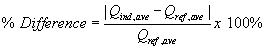(ii) To successfully pass this test, the percent difference calculated in Equation 14 of this paragraph (g)(3) shall not exceed 2 percent for each test run.

(4) Flow rate coefficient of variation measurement accuracy. (i) Using the flow rate coefficient of variation indicated by the candidate test sampler (%CVind), determine the accuracy of the reported coefficient of variation as:

Equation 15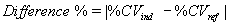(ii) To successfully pass this test, the absolute difference calculated in Equation 15 of this paragraph (g)(4) must not exceed 0.3 (CV%) for each test run.

(5) Ambient temperature measurement accuracy. (i) Calculate the absolute value of the difference between the mean ambient air temperature indicated by the test sampler and the mean ambient (chamber) air temperature measured with the ambient air temperature recorder as: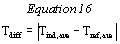Where: Tind,ave = The mean ambient air temperature indicated by the test sampler, °C; and Tref,ave = The mean ambient air temperature measured by the reference temperature instrument, °C.

(ii) The calculated temperature difference must be less than 2 °C for each test run.

(6) Sampler functionality. To pass the sampler functionality test, the following two conditions must both be met for each test run:

(i) The sampler must not shutdown during any portion of the 6-hour test.

(ii) An inspection of the downloaded data from the test sampler verifies that all the data are consistent with normal operation of the sampler.

[62 FR 38799, July 18, 1997, as amended at 71 FR 61291, Oct. 17, 2006]